### Three-dimensional coordinate geometry

The principal elements in solid geometry (3-dimensional geometry) are points, lines and planes. In the plane (2-dimensional geometry), the incidence' properties are very straightforward:

• two points determine a line
• two lines are parallel or meet at a unique point.

In 3-dimensional space, things are more complicated:

• two points still determine a line
• two lines meet or are parallel or are skew.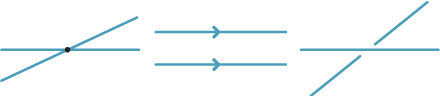To visualise a pair of skew lines, think of one as being behind' the other.

Three points which are not collinear determine a unique plane. But there are an infinite number of planes through a fixed line or three collinear points.

Two planes are parallel or meet in a line. Indeed, if two planes have a point in common, they must have a whole line of points in common (this is not obvious).

To set up a coordinate system for solid geometry, choose a plane $$0xy$$ and then fix axes $$0x$$ and $$0y$$ as in plane geometry.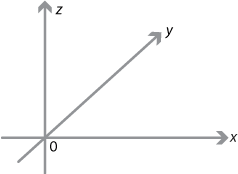The axis $$0z$$ is the normal to the plane $$0xy$$ through the origin. Usually we choose $$0z$$ to come out of the standard $$x$$–$$y$$ plane. (This is called a right-handed coordinate system and is very important when vectors are used — especially in applications in physics.)

Thus $$0x\perp 0y$$, $$0x\perp 0z$$ and $$0y\perp 0z$$.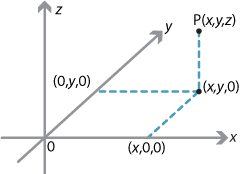Detailed description

Every point in 3-space is a certain distance $$z$$ above the $$x$$–$$y$$ plane (if $$z<0$$, the point is below). The points in the $$0xy$$ plane are given the coordinates $$(x,y,0)$$ and, in general, $$P$$ is $$(x,y,z)$$.

#### Midpoints and distance

Let $$P(x_1, y_1, z_1)$$ and $$Q(x_2, y_2, z_2)$$ be points in $$\mathbb {R}^3$$. Then:

• The midpoint of the line segment $$PQ$$ is $$M\Bigl(\dfrac{x_1+x_2}{2}, \dfrac{y_1 + y_2}{2}, \dfrac{z_1 + z_2}{2}\Bigr)$$.
• The distance between $$P$$ and $$Q$$ is $$\sqrt{(x_1-x_2)^2+(y_1-y_2)^2+(z_1-z_2)^2}$$.

So, for example, in the above diagram $$OP^2=x^2+y^2+z^2$$.

#### Lines in $$\mathbb{R}^3$$

To describe the points on a line, it is easiest to use a parameter which varies as a point moves along the line.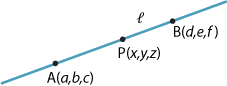Consider the line $$l$$ through the fixed points $$A(a,b,c)$$ and $$B(d,e,f)$$. Let $$P(x,y,z)$$ be any point on $$l$$. Then the vector $$(x-a,y-b,z-c)$$ is a scalar multiple $$t$$ of the vector $${(d-a,e-b,f-c)}$$. So

\begin{align*} x-a &= t(d-a)\\ y-b &= t(e-b)\\ z-c &= t(f-c) \end{align*} and thus \begin{align*} x &= td+(1-t)a\\ y &= te+(1-t)b\\ z &= tf+(1-t)c, \end{align*} for $$t\in \mathbb{R}$$. This is the parametric form for a line in $$\mathbb{R}^3$$. If $$t=0$$, then $$P=A$$ and, if $$t=1$$, then $$P=B$$.

We can obtain the Cartesian form for the line by eliminating $$t$$. It is

$\dfrac{x-a}{d-a} = \dfrac{y-b}{e-b} = \dfrac{z-c}{f-c}.$

Parametric forms for curves in $$\mathbb{R}^2$$ are discussed in the module Quadratics.

#### Planes in $$\mathbb{R}^3$$

There are three principal planes in $$\mathbb{R}^3$$. The $$x$$–$$y$$ plane, which contains the axes $$0x$$ and $$0y$$, is given by

$\Pi_z = \bigl\{\, (x,y,z)\in \mathbb{R}^3 \bigm| z=0 \,\bigr\}.$

The other two principal planes are $$x=0$$ and $$y=0$$.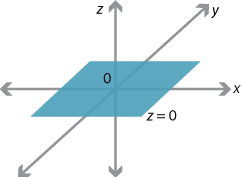Consider the set

$\Pi = \bigl\{\, (x,y,z)\in \mathbb{R}^3 \bigm| 3x+2y=6 \,\bigr\}.$

If $$z=0$$, then this restricts to a line in the $$x$$–$$y$$ plane.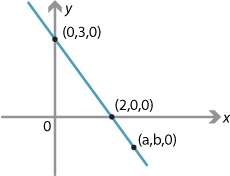However, if $$(a,b,0)$$ is any point on this line, then $$(a,b,z)\in\Pi$$ for any value of $$z$$. Thus $$\Pi$$ is a plane in $$\mathbb{R}^3$$. Indeed $$\Pi$$ is a plane parallel to the $$z$$-axis. In a similar way, $$3y+2z=6$$ is a plane parallel to the $$x$$-axis, and $$3x+2z=6$$ is a plane parallel to the $$y$$-axis.

Now consider the set

$\Pi = \bigl\{\, (x,y,z)\in \mathbb{R}^3 \bigm| 3x+2y+4z=12 \,\bigr\}.$

Given the previous examples, it should be no surprise that this is a plane. Clearly it passes through the points $$(4,0,0)$$, $$(0,6,0)$$ and $$(0,0,3)$$, so the plane has three intercepts!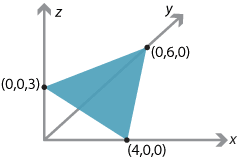The general equation for a plane is

$\Pi = \bigl\{\, (x,y,z)\in \mathbb{R}^3 \bigm| ax+by+cz=d \,\bigr\},$ where at least one of $$a,b,c$$ is non-zero.

So, in some ways, the general plane in $$\mathbb{R}^3$$ is analogous to the line in $$\mathbb{R}^2$$. To prove the above facts about planes, it is best to use vectors to represent the points in the plane.

Next page - Links forward - Quadratic equations and the conics